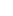Categories

Cheating at Perl

Perl code snippet cheat sheet.

Send files to recycle bin:

use Win32::FileOp qw(Recycle);
Recycle(@ARGV);

Parse out file path:

use File::Basename;

(\$name, \$path, \$suffix) = fileparse(\$item, '\.[^\.]*');

#where \$item is file with fullpath (perhaps derived from path::class

Split at last instance:

# Splitting at last instance of \
(\$a,\$b) = \$line =~ /(.*)\\(.*)/;

Split at first instance:

# Splitting at first instance of -
(\$a, \$b) = split(/\-/, \$line, 2);

Open file for overwriting:

open (OUTPUT, ">output.csv");

Open file for append writing:

open (OUTPUT, ">>output.csv");

Simple split:

(\$junk, \$use) = split (':', \$line);

use List::MoreUtils qw(uniq);

@uniq_list = uniq(@final);

Simple array sort:

@sorted = sort { \$a cmp \$b } @uniq_list;

Simple directory scan for files:

use Path::Class;

\$dir = dir('\\\\path\\TO\\dir');

while (my \$file = \$dir->next) {
next if \$file->is_dir();
push (@files, \$file);
}
foreach \$item (@files) {
print \$item;
}

Simple file copy:

use File::Copy qw(copy);

\$a = "path\to\source\file.txt";

\$b = "path\to\destination\file.txt";

print <code>copy \$a \$b</code>;

ODBC SQL Query:

use DBI;
use DBD::ODBC;

\$user="DBUSER";
\$host="DBSERVER";
\$start="DATABASE_NAME";

\$dsn = "dbi:ODBC:Driver={SQL Server};Server=\$host;Database=\$start"; \$dbh = DBI->connect(\$dsn, \$user, \$auth, { RaiseError => 1 });
#Example query below - simply lists databases in MS SQL
\$sql = qq/SELECT name FROM sys.databases/;
\$sth = \$dbh->prepare(\$sql);
\$sth->execute();

#Time to manipulate the return
while (@row= \$sth->fetchrow_array) {
unshift (@row, \$database);
#Print the data
print join("", @row), "\n";
#Push the data into an array
push (@databases, join("", @row), "\n");
}

\$line =~ s/^\s+//;

Remove trailing whitespace:

\$line =~ s/\s+\$//;

\$line =~ s/^\s*(.*?)\s*\$/\1/;

Replace multi whitespace with commas:

\$line =~ s/\s+/,/g;

Date (including yesterday’s date)

use DateTime;

&time;

sub time {
\$logdate = DateTime->now;
\$yesterday = DateTime->now->subtract( days =>  1 );
#Today's date:
(\$date, \$time) = split('T', \$logdate);
#Yesterday's date:
(\$date, \$time) = split('T', \$yesterday);
}